## 20170905

### The Third Way

This post was triggered by a brief exchange with Jan-Willem van Ree (on musescore) about the use of certain intervals in structured or otherwise constrained music. Having believed I had seen, long ago, examples of tone rows built exclusively from major and minor thirds (or their interval class equivalent, minor and major sixths), I’d formed the impression that either Webern or Berg had actively sought such sequences.

Dr van Ree kindly reminded me of the Berg Violin Concerto which includes the following run of eight successive thirds (abstracted and annotated below, with both note names and semitone interval classes between notes), and which finishes off with a triple whole tone tritone run. In the score’s introduction (Universal Edition Philharmonia Partituren #426), “F.S.” describes it as being the one from which the Concerto is made (presumably its prime ‘P0’ form - although it's not a strict 12 tone work).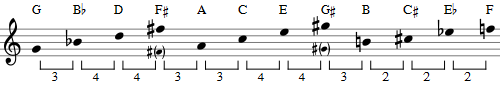Being the sort of thing I was looking for, the start of the sequence might be represented by the curved (red) path in the following transition-by-thirds (dark/light blue arrows for minor/major thirds respectively) diagram: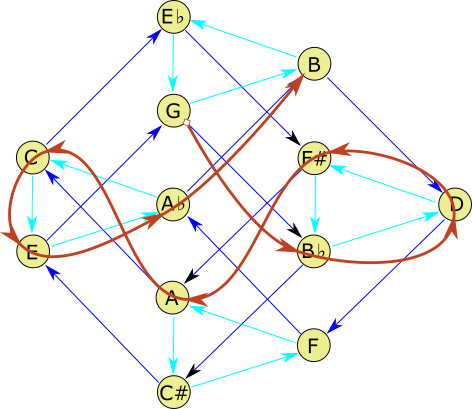But with this we may seek circuits, i.e. closed paths. For instance one might start at E, move to G, then B, then to D, to F, to A♭, to C, to E♭, to F#, to B♭, to C# and back to the starting E. That’s a circuit of eleven pitches, visiting each note only once. The one missed is the A. Is there any way to visit all 12, and end up where we started?

Unhappily not. There's no path which can complete such a circuit. You cannot find a path from any note back to itself visiting every other exactly once on the way.

You may, however, visit all notes exactly once – you just don’t get to return home. Here is one such path, again the curved one, starting at C and finishing at B: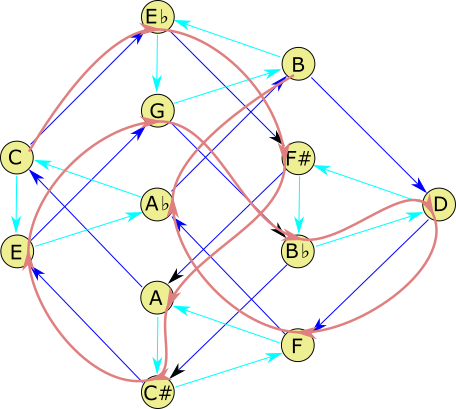The length of the route is 3+3+3+4+3+3+3+4+3+3+3 = 35. If the diagram would allow you to step 1 semitone then you could make it home from B to C (a nicely cadential leading tone) at 36.

There are four such paths (or 48, 12 each, if you care about your start position, but in pitch class world we really don’t). The jump patterns (and path lengths thereof) are:

1. 35 = 3+3+3+4+3+3+3+4+3+3+3(+1)
2. 37 = 4+3+3+4+3+3+3+4+3+3+4(-1)
3. 39 = 4+3+3+4+4+3+4+4+3+3+4(-3)
4. 41 = 4+4+3+4+4+3+4+4+3+4+4(-5)

The numbers in parentheses at the end are the extra steps you'd need to return to your start position. If you insist on upward jumps only then you must change the last three 'homing' jumps to +11, +9 and +7.

Completenesswise, journey number 4 also has a resolving flavour with its dominant/tonic termination. Journey 3 doesn’t, but the final jump home is at least another third, it’s just in the ‘wrong’ (with this diagram) direction.

Another representation of such circuits is by drawing them on the 12 hour pitch class clock:

where the modulo 12 labelling describes the pitch class visit-order – all of them starting from 0 (at 12 o’clock) and tracing the black lines strictly clockwise in their 3 or 4 ‘hourly’ jumps, except for that final jump, back to 0 (A and B being 10 and 11 respectively). You may click on the coloured areas to watch the traversals.

It will not have escaped your attention that, plotted this way, bilateral polygonal symmetry is apparent. Musically, this simply admits that their retrogrades and inversions are identical (descending thirds instead of ascending ones). The inverse of a minor third is a major sixth (3 + 9 = 12), and vice versa (4 + 8 = 12). The differences are all interval class 3 or 4. The third clock diagram is distinguished green only because, unlike the others, the closing leap happens to be in that very interval class (+9 ≡ –3, modulo 12).

But once we’ve admitted the equivalent interval classes of sixths and thirds, we may dispense with the arrowheads, since we may now move from node to node in either direction (major 3rd being equivalent to minor sixth, minor third to major sixth, either rising or falling). We may also take the opportunity to dispense with note names and abstract to pitch classes. The following transition diagram results: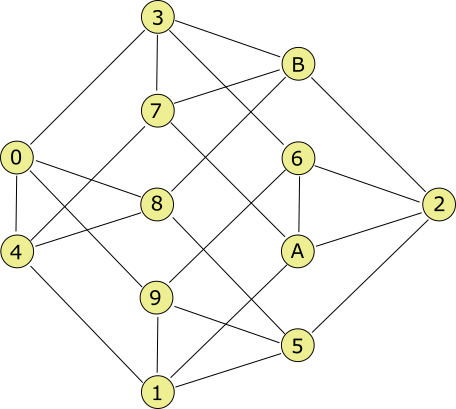Where the A and the B represent pitch classes 10 and 11 (not notes A and B!).

Now, since one may move in either direction, the possibilities for complete circuits (visiting all twelve pitch classes exactly once in a closed loop) would appear to dramatically increase. As in fact they have, to 252 in sheer numbers. But as far as the actual patterns of such loops are concerned, where (musical) transposition of a circuit is the same as starting at a different point, retrograde is circuiting in the opposite direction, and inversion as reflection, etc, it turns out that there are only 11 distinct patterns. In ‘alphabetical’ order, these are

1. 0362591A7B84 → +3+3-4+3+4+4-3-3+4-3-4(-4) ≡ +3+3+8+3+4+4+9+9+4+9+8(+8)
2. 0362A147B859 → +3+3-4-4+3+3+3+4-3-3+4(+3) ≡ +3+3+8+8+3+3+3+4+9+9+4(+3)
3. 0362A1958B74 → +3+3-4-4+3-4-4+3+3-4-3(-4) ≡ +3+3+8+8+3+8+8+3+3+8+9(+8)
4. 0362A7B84159 → +3+3-4-4-3+4-3-4-3+4+4(+3) ≡ +3+3+8+8+9+4+9+8+9+4+4(+3)
5. 0362B7A14859 → +3+3-4-3-4+3+3+3+4-3+4(+3) ≡ +3+3+8+9+8+3+3+3+4+9+4(+3)
6. 0362B847A159 → +3+3-4-3-3-4+3+3+3+4+4(+3) ≡ +3+3+8+9+9+8+3+3+3+4+4(+3)
7. 0369152A7B84 → +3+3+3+4+4-3-4-3+4-3-4(-4) ≡ +3+3+3+4+4+9+8+9+4+9+8(+8)
8. 036A1952B748 → +3+3+4+3-4-4-3-3-4-3+4(+4) ≡ +3+3+4+3+8+8+9+9+8+9+4(+4)
9. 036A259147B8 → +3+3+4+4+3+4+4+3+3+4-3(+4) ≡ +3+3+4+4+3+4+4+3+3+4+9(+4)
10. 037B26A19584 → +3+4+4+3+4+4+3-4-4+3-4(-4) ≡ +3+4+4+3+4+4+3+8+8+3+8(+8)
11. 037B2A691584 → +3+4+4+3-4-4+3+4+4+3-4(-4) ≡ +3+4+4+3+8+8+3+4+4+3+8(+8)

The above 'pitch class clock diagrams' show all 11 species of ‘dodecacircuits’ where every jump size is either ±3 or ±4 semitones. Musically, these steps are minor or major thirds (up or down) in pitch class (or, respectively, major or minor sixths, down or up). Of the 11 patterns, 10 turn up 24 times (rotations & reflections leaving their essential paths unaltered) and 1 turns up only 12 times (because it has two axes of symmetry) - the last shown, in dark blue. Thus accounting for the 252.

Two are coloured yellow; unlike the others, they are unsymmetric. The pair are, however, related – one being a reflection of the other (musical inversion) most easily perceived by flipping (say) the second one (0369152A7B84) in its horizontal (3 o’clock to 9 o’clock) axis (i.e. it cannot rotate into the other, only flip).

All others are symmetric – the brighter blue pair are so distinguished only because their single axis of symmetry happens to cut through an axis on whole hours (opposing dodecagonal vertices) rather than the majority whose axes of symmetry lie over half-hours (opposing dodecagonal edges). For instance the symmetry axis on 0362A147B859 is at 2 o’clock to 8 o’clock, but lies at 2:30 to 8:30 on 036A1952B748. That last one (number 8 in the above list, with the interval path +3+3+4+3-4-4-3-3-4-3+4 is depicted below: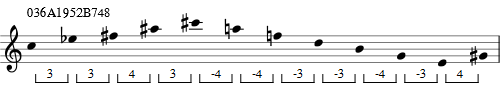And at the end it will take the step of a major third to start the row afresh.

Notice that the ninth dodecagon, labelled 036A259147B8 (the first one in the third row here) is our old friend from the quartet of ‘third-up-only circuiters’ from before, labelled 047A269158B3. It turns up here because its final jump back (of –3) is in the interval class allowed by our new bidirectional interval jumping rule. To see that this is indeed the case, move the first character of the earlier label to the front, thus 047A269158B3 → 47A269158B30 (equivalent to rotating the polygonal pattern by one ‘hour’). Whereupon you now subtract 4 (or, equivalently modulo 12, add 8) to each character of this rotated label to restart it at zero. Thus 47A269158B30 → 036A259147B8, precisely the label of the ninth one above.

The labels above are chosen because they are – alphabetically – the ‘lowest valued’ ones of the (generally 24) possible ‘pitch class path’ labellings for each shape, i.e. with the longest runs of minor third runs at the start, then the major thirds, minor sixths and major sixths. Consequently the polygons drawn are the ones determined by that label.